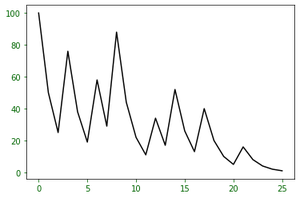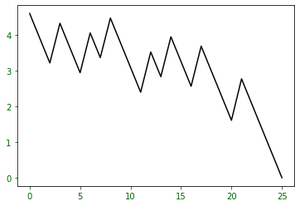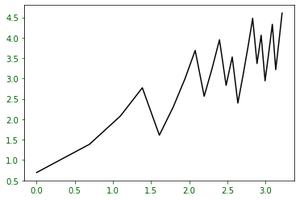Importance of the Collatz conjecture

• Last Updated : 23 Dec, 2021

Introduction :
The Collatz conjecture is an elusive problem in mathematics regarding the oneness of natural numbers when run through a specific function based on being odd or even, specifically starting that regardless of the initial number the series will eventually reach the number 1. The Collatz Conjecture has been an internationally popular problem in mathematical circles since the early part of the 20th century when the German mathematician Lothar Collatz is credited with the origination of the problem.

Description of “the conjecture” :
The conjecture statement states –
Take any natural number n. If n is even, divide it by 2 to get n/2, if n is odd multiply it by 3 and add 1 to obtain 3n+1. Repeat the process indefinitely. The conjecture is that no matter what number you start with, you will always eventually reach 1.

So for a natural number n, we can define the following function –

T(n) = n/2                              n≡0(mod2)
= (3*n) + 1                     n≡1(mod2)

Some Study :
The function’s progress over successive iteration while n>1 can be easily studied and visualized with the help of a graph. For this, we have this corresponding python code for simulating some runs and creating a plot.

Python3

 from matplotlib import pyplot as plt n = int(input()) x = [] x.append(n) while(n > 1):     if(n % 2 == 0):         n = n//2         x.append(n)         print(n)     else:         n = (3*n) + 1         x.append(n)         print(n) plt.plot(x, '-ok') plt.show()The above graph is for starting value of 100. You can try out the simulation code with some starting values of your own. As you can see the above graph is quite chaotic. There’s no distinct pattern other than it eventually converging to 1, As the values may become quite large very quickly. 100 takes 25 steps to converge, but others might take even more or less.

Let’s try out some more graphs, by graphing in a semi-log grid where the y-axis is logarithmic, the x-axis remains linear. The python code for the method is :

Python3

 from matplotlib import pyplot as plt import numpy as np      y = [] n = 100 y.append(n) while(n > 1):   if (n % 2 == 0):     n = n//2   else:     n = (3*n) + 1   y.append(n) x = range(0,len(y)) plt.plot(x,np.log(y)) plt.show()

The graph for the above code is:Now we can reverse the y-axis in another graphing methodology, to study the growth from 1 and create a plot as follows:Now to find some “patterns” on how many steps it takes for our input number to converge to 1, let’s change the x-axis of the above graphing method to log scale. The python code for the said is as follows:

Python3

 from matplotlib import pyplot as plt import numpy as np      y = [] n = 100 y.append(n) while(n > 1):   if (n % 2 == 0):     n = n//2   else:     n = (3*n) + 1   y.append(n) x = range(0,len(y)) plt.plot(np.log(x),np.log(y[::-1])) plt.show()

The graph for the above code is as shown below, where the y-axis is the collatz value in log and the x-axis is the reversed step order.Now with the above graphing method we can try and plot a bunch of different such series to study and compare the growth of each series.

My Personal Notes arrow_drop_up
Recommended Articles
Page :